# Practical Introduction to Time-Frequency Analysis

This example shows how to perform and interpret basic time-frequency signal analysis. In practical applications, many signals are nonstationary. This means that their frequency-domain representation (their spectrum) changes over time. The example discusses the advantages of using time-frequency techniques over frequency-domain or time-domain representations of a signal. It answers basic questions, such as: When is a particular frequency component present in my signal? How do I increase time or frequency resolution? How can I sharpen the spectrum of a component or extract a particular mode? How do I measure power in a time-frequency representation? How do I visualize the time-frequency information of my signal? How do I find intermittent interference within the frequency content of the signal of interest?

### Using Time-Frequency Analysis to Identify Numbers in a DTMF Signal

You can divide almost any time-varying signal into time intervals short enough that the signal is essentially stationary in each section. Time-frequency analysis is most commonly performed by segmenting a signal into those short periods and estimating the spectrum over sliding windows. The `pspectrum` function used with the `'spectrogram'` option computes an FFT-based spectral estimate over each sliding window and lets you visualize how the frequency content of the signal changes over time.

Consider the signaling system of a digital phone dial. The signals produced by such a system are known as dual-tone multi-frequency (DTMF) signals. The sound generated by each dialed number consists of the sum of two sinusoids $-$ or tones $-$ with frequencies taken from two mutually exclusive groups. Each pair of tones contains one frequency of the low group (697 Hz, 770 Hz, 852 Hz, or 941 Hz) and one frequency of the high group (1209 Hz, 1336 Hz, or 1477Hz) and represents a unique symbol. The following are the frequencies allocated to the buttons of a telephone pad:Generate a DTMF signal and listen to it.

```[tones, Fs] = helperDTMFToneGenerator(); p = audioplayer(tones,Fs,16); play(p)```

Listening to the signal, you can tell that a three-digit number was dialed. However, you cannot tell which number it was. Next, visualize the signal in time and in frequency domain over the 650 to 1500 Hz band. Set the `'Leakage'` parameter of the `pspectrum` function to 1 to use a rectangular window and improve frequency resolution.

```N = numel(tones); t = (0:N-1)/Fs; subplot(2,1,1) plot(1e3*t,tones) xlabel('Time (ms)') ylabel('Amplitude') title('DTMF Signal') subplot(2,1,2) pspectrum(tones,Fs,'Leakage',1,'FrequencyLimits',[650, 1500])```The time-domain plot of the signal confirms the presence of three bursts of energy, corresponding to three pushed buttons. To measure the length of the burst, you can take the pulse width of the RMS envelope.

```env = envelope(tones,80,'rms'); pulsewidth(env,Fs)```
```ans = 3×1 0.1041 0.1042 0.1047 ```
`title('Pulse Width of RMS Envelope')`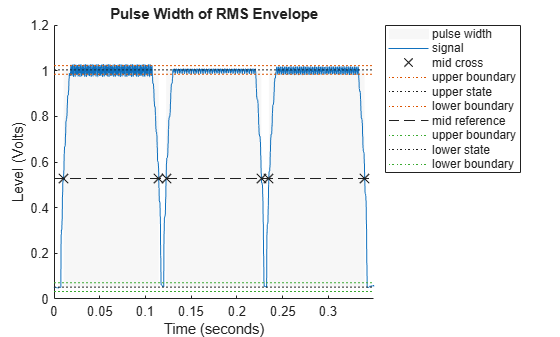Here you can see three pulses, each one approximately 100 milliseconds long. However, you cannot tell which numbers were dialed. A frequency-domain plot helps you figure this out because it shows the frequencies present in the signal.

Locate the frequency peaks by estimating the mean frequency in four different frequency bands.

```f = [meanfreq(tones,Fs,[700 800]), ... meanfreq(tones,Fs,[800 900]), ... meanfreq(tones,Fs,[900 1000]), ... meanfreq(tones,Fs,[1300 1400])]; round(f)```
```ans = 1×4 770 852 941 1336 ```

By matching the estimated frequencies to the diagram of the telephone pad, you can say that the dialed buttons were '5', '8', and '0'. However, the frequency-domain plot does not provide any type of time information that would allow you to figure out the order in which they were dialed. The combination could be '580','508','805','850', '085', or '058'. To solve this puzzle, use the pspectrum function to compute the spectrogram and observe how the frequency content of the signal varies with time.

Compute the spectrogram over the 650 to 1500 Hz band and remove content below the $-$10 dB power level to visualize only the main frequency components. To see the tone durations and their locations in time use 0% overlap.

```pspectrum(tones,Fs,'spectrogram','Leakage',1,'OverlapPercent',0, ... 'MinThreshold',-10,'FrequencyLimits',[650, 1500]);```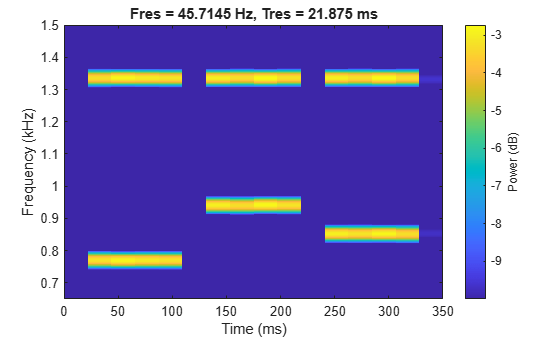The colors of the spectrogram encode frequency power levels. Yellow colors indicate frequency content with higher power; blue colors indicate frequency content with very low power. A strong yellow horizontal line indicates the existence of a tone at a particular frequency. The plot clearly shows the presence of a 1336 Hz tone in all three dialed digits, telling you that they are all on the second column of the keypad. From the plot you can see that the lowest frequency, 770 Hz, was dialed first. The highest frequency, 941 Hz, was next. The middle frequency, 852 Hz, came last. Hence, the dialed number was 508.

The `pspectrum` function divides a signal into segments. Longer segments provide better frequency resolution; shorter segments provide better time resolution. The segment lengths can be controlled using the `'FrequencyResolution'` and `'TimeResolution'` parameters. When no frequency resolution or time resolution values are specified, `pspectrum` attempts to find a good balance between time and frequency resolutions based on the input signal length.

Consider the following signal, sampled at 4 kHz, that consists of the trill portion of a Pacific blue whale song:

```load whaleTrill p = audioplayer(whaleTrill,Fs,16); play(p)```

The trill signal consists of a train of tonal pulses. Look at the time signal and the spectrogram obtained by `pspectrum` when no resolution is specified and when time resolution is set to 10 milliseconds. Set the `'Leakage'` parameter to 1 to use rectangular windows. Since we want to localize the time position of the pulses, set overlap percent to 0. Finally, use a `'MinThreshold'` of $-$60 dB to remove background noise from the spectrogram view.

```t = (0:length(whaleTrill)-1)/Fs; figure ax1 = subplot(3,1,1); plot(t,whaleTrill) ax2 = subplot(3,1,2); pspectrum(whaleTrill,Fs,'spectrogram','OverlapPercent',0, ... 'Leakage',1,'MinThreshold',-60) colorbar(ax2,'off') ax3 = subplot(3,1,3); pspectrum(whaleTrill,Fs,'spectrogram','OverlapPercent',0, ... 'Leakage',1,'MinThreshold',-60,'TimeResolution', 10e-3) colorbar(ax3,'off') linkaxes([ax1,ax2,ax3],'x')```The 47 milliseconds time resolution chosen by `pspectrum` is not small enough to localize all the trill pulses in the spectrogram. On the other hand, a time resolution of 10 milliseconds is enough to localize each trill pulse in time. This becomes even clearer if we zoom into a few pulses:

`xlim([0.3 0.55])`Now load a signal that consists of an echolocation pulse emitted by a big brown bat (Eptesicus fuscus). The signal is measured with a sampling interval of 7 microseconds. Analyze the spectrogram of the signal.

```load batsignal Fs = 1/DT; figure pspectrum(batsignal,Fs,'spectrogram')```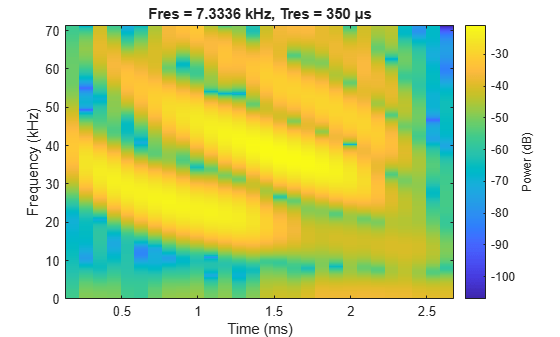The spectrogram with default parameter values shows four coarse time-frequency ridges. Reduce the frequency resolution value to 3 kHz to get more details on the frequency variation of each ridge.

`pspectrum(batsignal,Fs,'spectrogram','FrequencyResolution',3e3)`Observe that now the frequency ridges are better localized in frequency. However, since frequency and time resolution are inversely proportional, the time resolution of the spectrogram is considerably smaller. Set an overlap of 99% to smooth out the time windows. Use a `'MinThreshold'` of $-$60 dB to remove unwanted background content.

```pspectrum(batsignal,Fs,'spectrogram','FrequencyResolution',3e3, ... 'OverlapPercent',99,'MinTHreshold',-60)```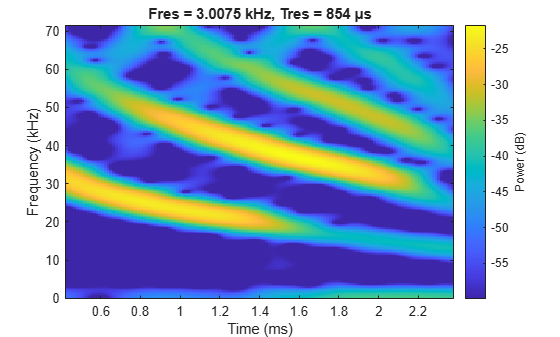The new settings yield a spectrogram that clearly shows the four frequency ridges of the echolocation signal.

### Time-Frequency Reassignment

Even though we have been able to identify four frequency ridges, we can still see that each ridge is spread over several adjacent frequency bins. This is due to the leakage of the windowing method used in both time and frequency.

The `pspectrum` function is capable of estimating the center of energy for each spectral estimate in both time and frequency. If you reassign the energy of each estimate to the bin closest to the new time and frequency centers, you can correct for some of the leakage of the window. You can do this by using the `'Reassign'` parameter. Setting this parameter to `true` computes the reassigned spectrogram of the signal.

```pspectrum(batsignal,Fs,'spectrogram','FrequencyResolution',3e3, ... 'OverlapPercent',99,'MinTHreshold',-60,'Reassign',true)```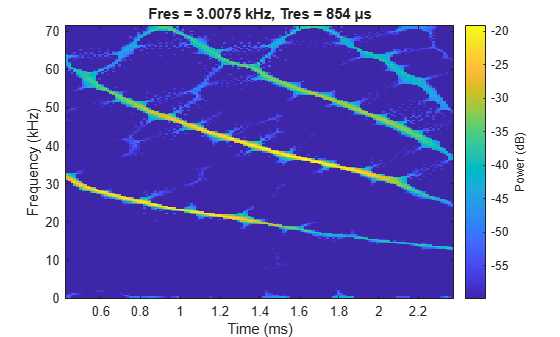Now the frequency ridges are much sharper and better localized in time. You can also localize the signal energy using the function `fsst`, which is discussed in the next section.

### Reconstructing a Time-Frequency Ridge

Consider the following recording, consisting of a chirp signal whose frequency decreases over time and a final splat sound.

```load splat p = audioplayer(y,Fs,16); play(p) pspectrum(y,Fs,'spectrogram')```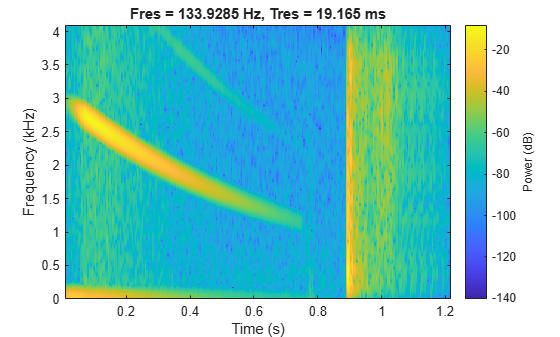Let us reconstruct a portion of the "splat" sound by extracting a ridge in the time-frequency plane. We use `fsst` to sharpen the spectrum of a noisy version the splat signal, `tfridge` to identify the ridge of the chirp sound, and `ifsst` to reconstruct the chirp. The process denoises the reconstructed signal.

Add Gaussian noise to the chirp portion of the "splat" sound. The added noise simulates an audio recording taken with an inexpensive microphone. Examine the time-frequency spectral content.

```rng('default') t = (0:length(y)-1)/Fs; yNoise = y + 0.1*randn(size(y)); yChirp = yNoise(t<0.35); pspectrum(yChirp,Fs,'spectrogram','MinThreshold',-70)```Sharpen the spectrum using the Fourier synchrosqueezed transform, `fsst`. `fsst` localizes energy in the time-frequency plane by reassigning energy in frequency for a fixed time. Compute and plot the synchrosqueezed transform of the noisy chirp.

`fsst(yChirp,Fs,'yaxis')`The chirp appears as a localized ridge in the time-frequency plane. Identify the ridge using `tfridge`. Plot the ridge along with the transform.

```[sst,f] = fsst(yChirp,Fs); [fridge, iridge] = tfridge(sst,f,10); helperPlotRidge(yChirp,Fs,fridge);```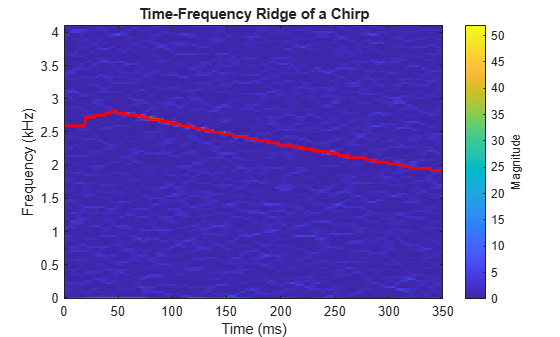Next, reconstruct the chirp signal using the ridge index vector `iridge`. Include one bin on each side of the ridge. Plot the spectrogram of the reconstructed signal.

```yrec = ifsst(sst,kaiser(256,10),iridge,'NumFrequencyBins',1); pspectrum(yrec,Fs,'spectrogram','MinThreshold',-70)```Reconstructing the ridge has removed noise from the signal. Play the noisy and denoised signals consecutively to hear the difference.

```p = audioplayer([yChirp;zeros(size(yChirp));yrec],Fs,16); play(p);```

### Measuring Power

Consider a complex linear frequency modulated (LFM) pulse, which is a common radar waveform. Compute the spectrogram of the signal using a time resolution of 1.27 microseconds and 90% overlap.

```Fs = 1e8; bw = 60e6; t = 0:1/Fs:10e-6; IComp = chirp(t,-bw/2,t(end), bw/2,'linear',90)+0.15*randn(size(t)); QComp = chirp(t,-bw/2,t(end), bw/2,'linear',0) +0.15*randn(size(t)); IQData = IComp + 1i*QComp; segmentLength = 128; pspectrum(IQData,Fs,'spectrogram','TimeResolution',1.27e-6,'OverlapPercent',90)```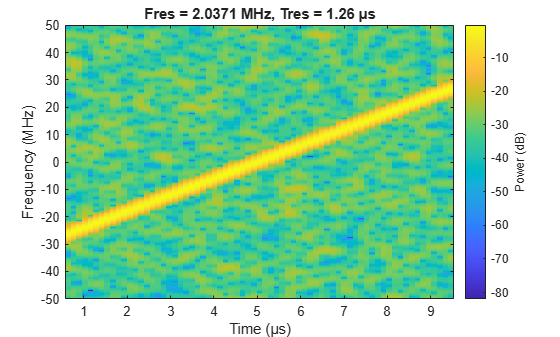The parameters used to compute the spectrogram give a clear time-frequency representation of the LFM signal. `pspectrum` computes a power spectrogram, this means that the color values correspond to true power levels in dB. The color bar shows that the power level of the signal is around $-$4 dB.

### Logarithmic Frequency Scale Visualization

In certain applications, it may be preferable to visualize the spectrogram of a signal on a logarithmic frequency scale. You can achieve this by changing the `YScale` property of the y-axis. For example, consider a logarithmic chirp sampled at 1 kHz. The frequency of the chirp increases from 10 Hz to 400 Hz in 10 seconds.

```Fs = 1e3; t = 0:1/Fs:10; fo = 10; f1 = 400; y = chirp(t,fo,10,f1,'logarithmic'); pspectrum(y,Fs,'spectrogram','FrequencyResolution',1, ... 'OverlapPercent',90,'Leakage',0.85,'FrequencyLimits',[1 Fs/2])```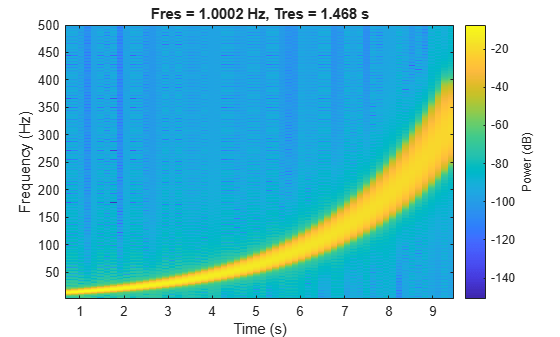The spectrogram of the chirp becomes a straight line when the frequency scale is logarithmic.

```ax = gca; ax.YScale = 'log';```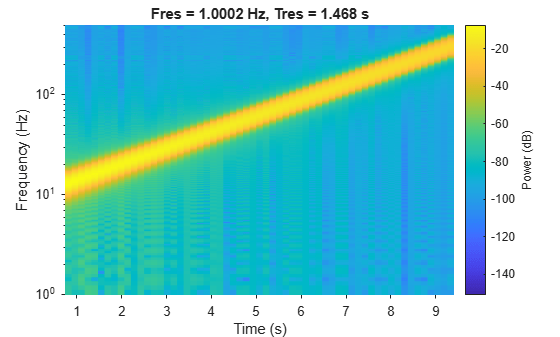### Three-Dimensional Waterfall Visualization

With the `view` command, you can visualize the spectrogram of a signal as a three-dimensional waterfall plot. You can also change the display colors with the `colormap` function.

```Fs = 10e3; t = 0:1/Fs:2; x1 = vco(sawtooth(2*pi*t,0.5),[0.1 0.4]*Fs,Fs); pspectrum(x1,Fs,'spectrogram','Leakage',0.8)```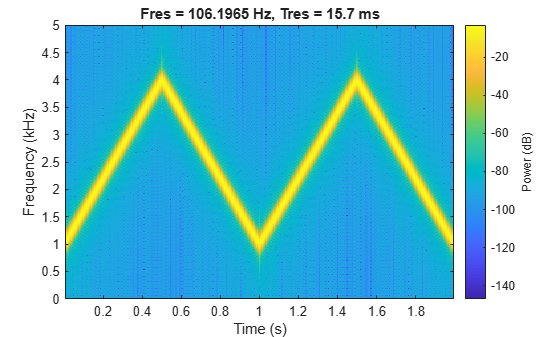```view(-45,65) colormap bone```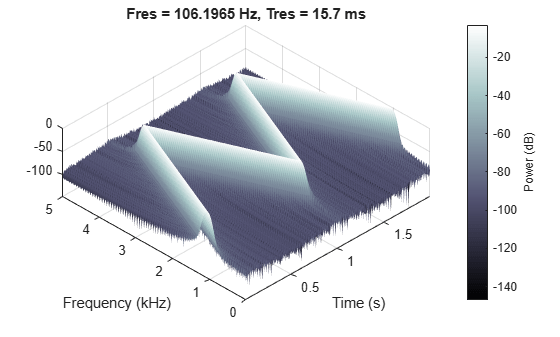### Finding Interferences Using Persistence Spectrum

The persistence spectrum of a signal is a time-frequency view that shows the percentage of the time that a given frequency is present in a signal. The persistence spectrum is a histogram in power-frequency space. The longer a particular frequency persists in a signal as the signal evolves, the higher its time percentage and thus the brighter or "hotter" its color in the display. Use the persistence spectrum to identify signals hidden in other signals.

Consider an interference narrowband signal embedded within a broadband signal. Generate a chirp sampled at 1 kHz for 500 seconds. The frequency of the chirp increases from 180 Hz to 220 Hz during the measurement.

```fs = 1000; t = (0:1/fs:500)'; x = chirp(t,180,t(end),220) + 0.15*randn(size(t));```

The signal also contains a 210 Hz interference, with an amplitude of 0.05, that is present only for 1/6 of the total signal duration.

```idx = floor(length(x)/6); x(1:idx) = x(1:idx) + 0.05*cos(2*pi*t(1:idx)*210);```

Compute the power spectrum of the signal over the 100 to 290 Hz interval. The weak sinusoid is obscured by the chirp.

`pspectrum(x,fs,'FrequencyLimits',[100 290])`Compute the persistence spectrum of the signal. Now both signal components are clearly visible.

```figure colormap parula pspectrum(x,fs,'persistence','FrequencyLimits',[100 290],'TimeResolution',1)```### Conclusions

In this example, you learned how to perform time-frequency analysis using the pspectrum function and how to interpret spectrogram data and power levels. You learned how to change time and frequency resolution to improve your understanding of signal and how to sharpen spectra and extract time-frequency ridges using `fsst`, `ifsst`, and `tfridge`. You learned how to configure the spectrogram plot to get a logarithmic frequency scale and three-dimensional visualization. Finally, you learned how to find interference signals by computing a persistence spectrum.

### Appendix

The following helper functions are used in this example.